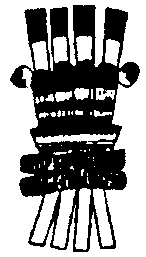# The Kabbalah and the year of BirthWe will now continue with mathematics our whole life unfolds with mathematics. The

Law of Karma develops with numbers.

1.-EXAMPLE: Eduardo de Portillo:

Date of birth: 1932.

To the year of birth, the same year is added.

1932

1

9

3

2

1947 = 1 + 9 + 4 + 7 = 21 (see Arcanum number 21)

21 = 2 + 1 = 3 (see Arcanum number 3)

1947

1

9

4

7

1968 = 1 + 9 + 6 + 8 = 24 (see Arcanum number 24)

24 2 + 4 = 6 the Lover. He met his fiancee in 1968.

1968

1

9

6

8

1992 = 1 + 9 + 9 + 2 = 21 (see Arcanum number 21).

21 = 2 + 1 = 3 marks a spiritual and economic triumph. That year will not be forgotten.

2.- EXAMPLE: Arturo Aguirre: Date of birth: 1937.

1937

1

9

3

7

1957 = 1 + 9 + 5 + 7 = 22 = 2 + 2 = 4: Success in the material plane.

1957

1

9

5

7

1979=1 + 9 + 7 + 9 = 26 = 2 + 6 = 8

8: Tests and suffering; to have the patience of Job.

3.- EXAMPLE: Luis Perez, year: 1920.

1920

1

9

2

0

1932= 1 + 9 + 3 + 2 = 15 => 1 + 5 = 6

6: Affliction of the heart, feeling; the 6 is related with the moral aspect that year his father

died, and this was very grave.

1932

1

9

3

2

1947 = 1 + 9 + 4 + 7 = 21 => 2 + 1 = 3

3: Material and spiritual production.

1947

1

9

4

7

1968 = 1 + 9 + 6 + 8 = 24: The Weaver, he became trapped in his own web; sufferings

which he had not left behind.

24 = 2 + 4 = 6: The Lover.

4.- EXAMPLE:Nicholas Naranjo, year: 1946.

1946

1

9

4

6

1966 = 1 + 9 + 6 + 6 = 22 => 2 + 2 = 4

4: Success because he encountered Gnosis.

1966

1

9

6

6

1988 = 1 + 9 + 8 + 8 = 26 => 2 + 6 = 8

8: He will continue working with success.